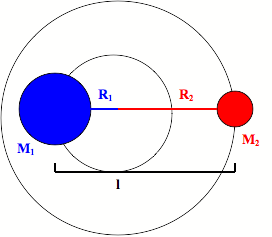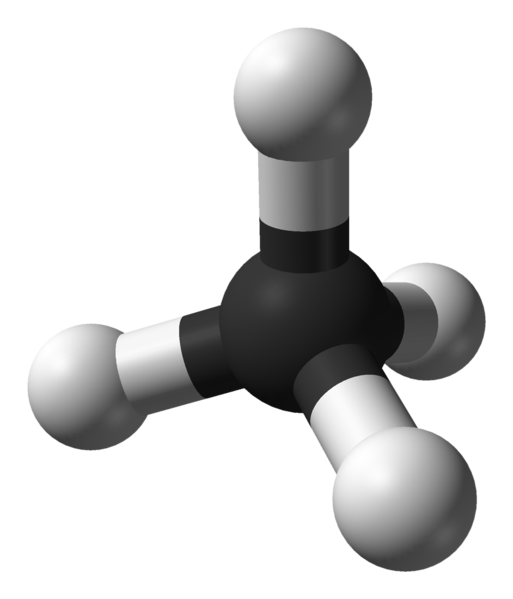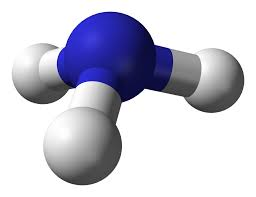# 1.10: Microwave Spectroscopy

$$\newcommand{\vecs}{\overset { \rightharpoonup} {\mathbf{#1}} }$$ $$\newcommand{\vecd}{\overset{-\!-\!\rightharpoonup}{\vphantom{a}\smash {#1}}}$$$$\newcommand{\id}{\mathrm{id}}$$ $$\newcommand{\Span}{\mathrm{span}}$$ $$\newcommand{\kernel}{\mathrm{null}\,}$$ $$\newcommand{\range}{\mathrm{range}\,}$$ $$\newcommand{\RealPart}{\mathrm{Re}}$$ $$\newcommand{\ImaginaryPart}{\mathrm{Im}}$$ $$\newcommand{\Argument}{\mathrm{Arg}}$$ $$\newcommand{\norm}{\| #1 \|}$$ $$\newcommand{\inner}{\langle #1, #2 \rangle}$$ $$\newcommand{\Span}{\mathrm{span}}$$ $$\newcommand{\id}{\mathrm{id}}$$ $$\newcommand{\Span}{\mathrm{span}}$$ $$\newcommand{\kernel}{\mathrm{null}\,}$$ $$\newcommand{\range}{\mathrm{range}\,}$$ $$\newcommand{\RealPart}{\mathrm{Re}}$$ $$\newcommand{\ImaginaryPart}{\mathrm{Im}}$$ $$\newcommand{\Argument}{\mathrm{Arg}}$$ $$\newcommand{\norm}{\| #1 \|}$$ $$\newcommand{\inner}{\langle #1, #2 \rangle}$$ $$\newcommand{\Span}{\mathrm{span}}$$$$\newcommand{\AA}{\unicode[.8,0]{x212B}}$$

Microwave rotational spectroscopy uses microwave radiation to measure the energies of rotational transitions for molecules in the gas phase. It accomplishes this through the interaction of the electric dipole moment of the molecules with the electromagnetic field of the exciting microwave photon.

## Introduction

To probe the pure rotational transitions for molecules, scientists use microwave rotational spectroscopy. This spectroscopy utilizes photons in the microwave range to cause transitions between the quantum rotational energy levels of a gas molecule. The reason why the sample must be in the gas phase is due to intermolecular interactions hindering rotations in the liquid and solid phases of the molecule. For microwave spectroscopy, molecules can be broken down into 5 categories based on their shape and the inertia around their 3 orthogonal rotational axes. These 5 categories include diatomic molecules, linear molecules, spherical tops, symmetric tops and asymmetric tops.

## Classical Mechanics

The Hamiltonian solution to the rigid rotor is

$H = T$

since,

$H = T + V$

Where $$T$$ is kinetic energy and $$V$$ is potential energy. Potential energy, $$V$$, is 0 because there is no resistance to the rotation (similar to a particle in a box model).

Since $$H = T$$, we can also say that:

${T = }\dfrac{1}{2}\sum{m_{i}v_{i}^2}$

However, we have to determine $$v_i$$ in terms of rotation since we are dealing with rotation. Since,

$\omega = \dfrac{v}{r}$

where $$\omega$$ = angular velocity, we can say that:

$v_{i} = \omega{X}r_{i}$

Thus we can rewrite the T equation as:

$T = \dfrac{1}{2}\sum{m_{i}v_{i}\left(\omega{X}r_{i}\right)}$

Since $$\omega$$ is a scalar constant, we can rewrite the T equation as:

$T = \dfrac{\omega}{2}\sum{m_{i}\left(v_{i}{X}r_{i}\right)} = \dfrac{\omega}{2}\sum{l_{i}} = \omega\dfrac{L}{2}$

where $$l_i$$ is the angular momentum of the ith particle, and L is the angular momentum of the entire system. Also, we know from physics that,

$L = I\omega$

where I is the moment of inertia of the rigid body relative to the axis of rotation. We can rewrite the T equation as,

$T = \omega\dfrac{{I}\omega}{2} = \dfrac{1}{2}{I}\omega^2$

## Quantum Mechanics

The internal Hamiltonian, H, is:

$H = \dfrac{i^{2}\hbar^{2}}{2I}$

and the Schrödinger Equation for rigid rotor is:

$\dfrac{i^{2}\hbar^{2}}{2I}\psi = E\psi$

Thus, we get:

$E_n = \dfrac{J(J+1)h^2}{8\pi^2I}$

where $$J$$ is a rotational quantum number and $$\hbar$$ is the reduced Planck's constant. However, if we let:

$B = \dfrac {h}{8 \pi^2I}$

where $$B$$ is a rotational constant, then we can substitute it into the $$E_n$$ equation and get:

$E_{n} = J(J+1)Bh$

Considering the transition energy between two energy levels, the difference is a multiple of 2. That is, from $$J = 0$$ to $$J = 1$$, the $$\Delta{E_{0 \rightarrow 1}}$$ is 2Bh and from J = 1 to J = 2, the $$\Delta{E}_{1 \rightarrow 2}$$ is 4Bh.Figure $$\PageIndex{1}$$: Energy levels and line positions calculated in the rigid rotor approximation. This diagram illustrates how transitions between the rotational energy levels of molecules map onto the energies at which these transitions are observed during laboratory experiments. (CC CS-BY 3.0; Nnrw).

## Theory

When a gas molecule is irradiated with microwave radiation, a photon can be absorbed through the interaction of the photon’s electronic field with the electrons in the molecules. For the microwave region this energy absorption is in the range needed to cause transitions between rotational states of the molecule. However, only molecules with a permanent dipole that changes upon rotation can be investigated using microwave spectroscopy. This is due to the fact that their must be a charge difference across the molecule for the oscillating electric field of the photon to impart a torque upon the molecule around an axis that is perpendicular to this dipole and that passes through the molecules center of mass.

This interaction can be expressed by the transition dipole moment for the transition between two rotational states

$\text{Probability of Transition}=\int \psi_{rot}(F)\hat\mu \psi_{rot}(I)d\tau$

Where Ψrot(F) is the complex conjugate of the wave function for the final rotational state, Ψrot(I) is the wave function of the initial rotational state , and μ is the dipole moment operator with Cartesian coordinates of μx, μy, μz. For this integral to be nonzero the integrand must be an even function. This is due to the fact that any odd function integrated from negative infinity to positive infinity, or any other symmetric limits, is always zero.

In addition to the constraints imposed by the transition moment integral, transitions between rotational states are also limited by the nature of the photon itself. A photon contains one unit of angular momentum, so when it interacts with a molecule it can only impart one unit of angular momentum to the molecule. This leads to the selection rule that a transition can only occur between rotational energy levels that are only one quantum rotation level (J) away from another1.

$\Delta\textrm{J}=\pm 1$

The transition moment integral and the selection rule for rotational transitions tell if a transition from one rotational state to another is allowed. However, what these do not take into account is whether or not the state being transitioned from is actually populated, meaning that the molecule is in that energy state. This leads to the concept of the Boltzmann distribution of states. The Boltzmann distribution is a statistical distribution of energy states for an ensemble of molecules based on the temperature of the sample2.

$\dfrac{n_J}{n_0} = \dfrac{e^{(-E_{rot}(J)/RT)}}{\sum_{J=1}^{J=n} e^{(-E_{rot}(J)/RT)}}$

where Erot(J) is the molar energy of the J rotational energy state of the molecule,

• R is the gas constant,
• T is the temperature of the sample.
• n(J) is the number of molecules in the J rotational level, and
• n0 is the total number of molecules in the sample.

This distribution of energy states is the main contributing factor for the observed absorption intensity distributions seen in the microwave spectrum. This distribution makes it so that the absorption peaks that correspond to the transition from the energy state with the largest population based on the Boltzmann equation will have the largest absorption peak, with the peaks on either side steadily decreasing.

## Degrees of Freedom

A molecule can have three types of degrees of freedom and a total of 3N degrees of freedom, where N equals the number of atoms in the molecule. These degrees of freedom can be broken down into 3 categories3.

• Translational: These are the simplest of the degrees of freedom. These entail the movement of the entire molecule’s center of mass. This movement can be completely described by three orthogonal vectors and thus contains 3 degrees of freedom.
• Rotational: These are rotations around the center of mass of the molecule and like the translational movement they can be completely described by three orthogonal vectors. This again means that this category contains only 3 degrees of freedom. However, in the case of a linear molecule only two degrees of freedom are present due to the rotation along the bonds in the molecule having a negligible inertia.
• Vibrational: These are any other types of movement not assigned to rotational or translational movement and thus there are 3N – 6 degrees of vibrational freedom for a nonlinear molecule and 3N – 5 for a linear molecule. These vibrations include bending, stretching, wagging and many other aptly named internal movements of a molecule. These various vibrations arise due to the numerous combinations of different stretches, contractions, and bends that can occur between the bonds of atoms in the molecule.

Each of these degrees of freedom is able to store energy. However, In the case of rotational and vibrational degrees of freedom, energy can only be stored in discrete amounts. This is due to the quantized break down of energy levels in a molecule described by quantum mechanics. In the case of rotations the energy stored is dependent on the rotational inertia of the gas along with the corresponding quantum number describing the energy level.

## Rotational Symmetries

To analyze molecules for rotational spectroscopy, we can break molecules down into 5 categories based on their shapes and their moments of inertia around their 3 orthogonal rotational axes:4

1. Diatomic Molecules
2. Linear Molecules
3. Spherical Tops
4. Symmetrical Tops
5. Asymmetrical Tops

### Diatomic Molecules

The rotations of a diatomic molecule can be modeled as a rigid rotor. This rigid rotor model has two masses attached to each other with a fixed distance between the two masses.It has an inertia (I) that is equal to the square of the fixed distance between the two masses multiplied by the reduced mass of the rigid rotor.

$\large I_e= \mu r_e^2$

$\large \mu=\dfrac{m_1 m_2} {m_1+m_2}$

Using quantum mechanical calculations it can be shown that the energy levels of the rigid rotator depend on the inertia of the rigid rotator and the quantum rotational number J2.

$E(J) = B_e J(J+1)$

$B_e = \dfrac{h}{8 \pi^2 cI_e}$

However, this rigid rotor model fails to take into account that bonds do not act like a rod with a fixed distance, but like a spring. This means that as the angular velocity of the molecule increases so does the distance between the atoms. This leads us to the nonrigid rotor model in which a centrifugal distortion term ($$D_e$$) is added to the energy equation to account for this stretching during rotation.

$E(J)(cm^{-1}) = B_e J(J+1) – D_e J^2(J+1)^2$

This means that for a diatomic molecule the transitional energy between two rotational states equals

$E=B_e[J'(J'+1)-J''(J''+1)]-D_e[J'^2(J'+1)^2-J''^2(J'+1)^2]\label{8}$

Where J’ is the quantum number of the final rotational energy state and J’’ is the quantum number of the initial rotational energy state. Using the selection rule of $$\Delta{J}= \pm 1$$ the spacing between peaks in the microwave absorption spectrum of a diatomic molecule will equal

$E_R =(2B_e-4D_e)+(2B_e-12D_e){J}''-4D_e J''^3$

### Linear Molecules

Linear molecules behave in the same way as diatomic molecules when it comes to rotations. For this reason they can be modeled as a non-rigid rotor just like diatomic molecules. This means that linear molecule have the same equation for their rotational energy levels. The only difference is there are now more masses along the rotor. This means that the inertia is now the sum of the distance between each mass and the center of mass of the rotor multiplied by the square of the distance between them2.

$\large I_e=\sum_{j=1}^{n} m_j r_{ej}^2$

Where mj is the mass of the jth mass on the rotor and rej is the equilibrium distance between the jth mass and the center of mass of the rotor.

### Spherical Tops

Spherical tops are molecules in which all three orthogonal rotations have equal inertia and they are highly symmetrical. This means that the molecule has no dipole and for this reason spherical tops do not give a microwave rotational spectrum.Figure $$\PageIndex{2}$$: Geometrical example of a spherical top

Examples:### Symmetrical Tops

Symmetrical tops are molecules with two rotational axes that have the same inertia and one unique rotational axis with a different inertia. Symmetrical tops can be divided into two categories based on the relationship between the inertia of the unique axis and the inertia of the two axes with equivalent inertia. If the unique rotational axis has a greater inertia than the degenerate axes the molecule is called an oblate symmetrical top. If the unique rotational axis has a lower inertia than the degenerate axes the molecule is called a prolate symmetrical top. For simplification think of these two categories as either frisbees for oblate tops or footballs for prolate tops.Figure $$\PageIndex{3}$$: Symmetric Tops: (Left) Geometrical example of an oblate top and (right) a prolate top. Images used with permission from Wikipedia.com.Figure $$\PageIndex{4}$$: Examples of symmetric tops. Benzene (oblate) XeF4 (oblate) ClCH3 (prolate) NH3 (prolate)

In the case of linear molecules there is one degenerate rotational axis which in turn has a single rotational constant. With symmetrical tops now there is one unique axis and two degenerate axes. This means an additional rotational constant is needed to describe the energy levels of a symmetrical top. In addition to the rotational constant an additional quantum number must be introduced to describe the rotational energy levels of the symmetric top. These two additions give us the following rotational energy levels of a prolate and oblate symmetric top

$E_{(J,K)}(cm^{-1})=B_e*J(J+1)+(A_e-B_e)K^2$

Where Be is the rotational constant of the unique axis, Ae is the rotational constant of the degenerate axes, $$J$$ is the total rotational angular momentum quantum number and K is the quantum number that represents the portion of the total angular momentum that lies along the unique rotational axis. This leads to the property that $$K$$ is always equal to or less than $$J$$. Thus we get the two selection rules for symmetric tops

$\Delta J = 0, \pm1$

$\Delta K=0$

when $$K\neq 0$$

$\Delta J = \pm1$

$\Delta K=0$

when $$K=0$$

However, like the rigid rotor approximation for linear molecules, we must also take into account the elasticity of the bonds in symmetric tops. Therefore, in a similar manner to the rigid rotor we add a centrifugal coupling term, but this time we have one for each quantum number and one for the coupling between the two.

$E_{(J,K)}(cm^{-1})=B_e J(J+1)-D_{eJ} J^2(J+1)^2+(A_e-B_e)*K^2 \label{13}$

$-D_{ek} K^4-D_{ejk} J(J +1)K^2 \label{14}$

### Asymmetrical Tops

Asymmetrical tops have three orthogonal rotational axes that all have different moments of inertia and most molecules fall into this category. Unlike linear molecules and symmetric tops these types of molecules do not have a simplified energy equation to determine the energy levels of the rotations. These types of molecules do not follow a specific pattern and usually have very complex microwave spectra.Figure $$\PageIndex{5}$$: Molecular examples of asymmetrical tops. (left) water and (right) acetone